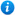20% OFF MEMBERSHIP THIS WEEKEND - Enter promo code MEM20 for 20% off* Watermarks do not appear on purchased resources

Preview resource: Click slides to enlarge

# Fractions of Amounts - Year 3

KS2 Maths Teaching Resources: Fractions of Amounts - Year 3

Year 3 maths programme of study - Number - fractions:

• recognise, find and write fractions of a discrete set of objects: unit fractions and non-unit fractions with small denominators
• recognise and use fractions as numbers: unit fractions and non-unit fractions with small denominators
• solve problems that involve all of the above

In this teaching resource, pupils understand that a fraction is one whole number divided by another, e.g. ¾ is 3 ÷ 4. They will practise finding fractions of a number or shape - such as 3/5, 1/4, 2/6. 'Fractions of Amounts - Year 3' is an ideal teaching aid to use in a lesson covering the year 3 curriculum objectives listed above.

This engaging 96 slide PowerPoint presentation and 14 worksheets is suitable to use for all abilities. Content includes:

• What are fractions explanation
• Recognising fractions activity and 2 accompanying worksheets
• Finding fractions of lollies and sheep activity
• Finding fractions of chicks activity
• Finding unit fractions of amounts explanation
• Circle a fraction of the objects activity and worksheet
• Unit fraction ladybird activity and worksheet
• Unit fractions of numbers activity and worksheet
• Fractions of measure activity
• Fractions code cracker activity and worksheet
• Finding non-unit fractions of amounts explanation
• Finding non-unit fractions of numbers activity and 2 worksheets
• Finding fractions of amounts fruit activity and worksheet
• Fractions word problems
• A link to a fractions of numbers game
• 3 further worksheets

'Fractions of Amounts - Year 3' is completely editable so that teachers can adapt the resource to suit their individual teaching needs.

To preview 'Fractions of Amounts - Year 3' in full please click on the PowerPoint images above.

Our Price : £3.99 / 4 Credits## Get this resource as part of a bundle and save up to 78%## Year 3 Fractions Bundle

£7.99## Year 3 Home Learning Pack 2

£13.99

By continuing to use the site, you agree to the use of cookies. You can change this and find out more by following this link